# Decide whether each chemical reaction in the table below is an oxidation-reduction ("redox") reaction. If the...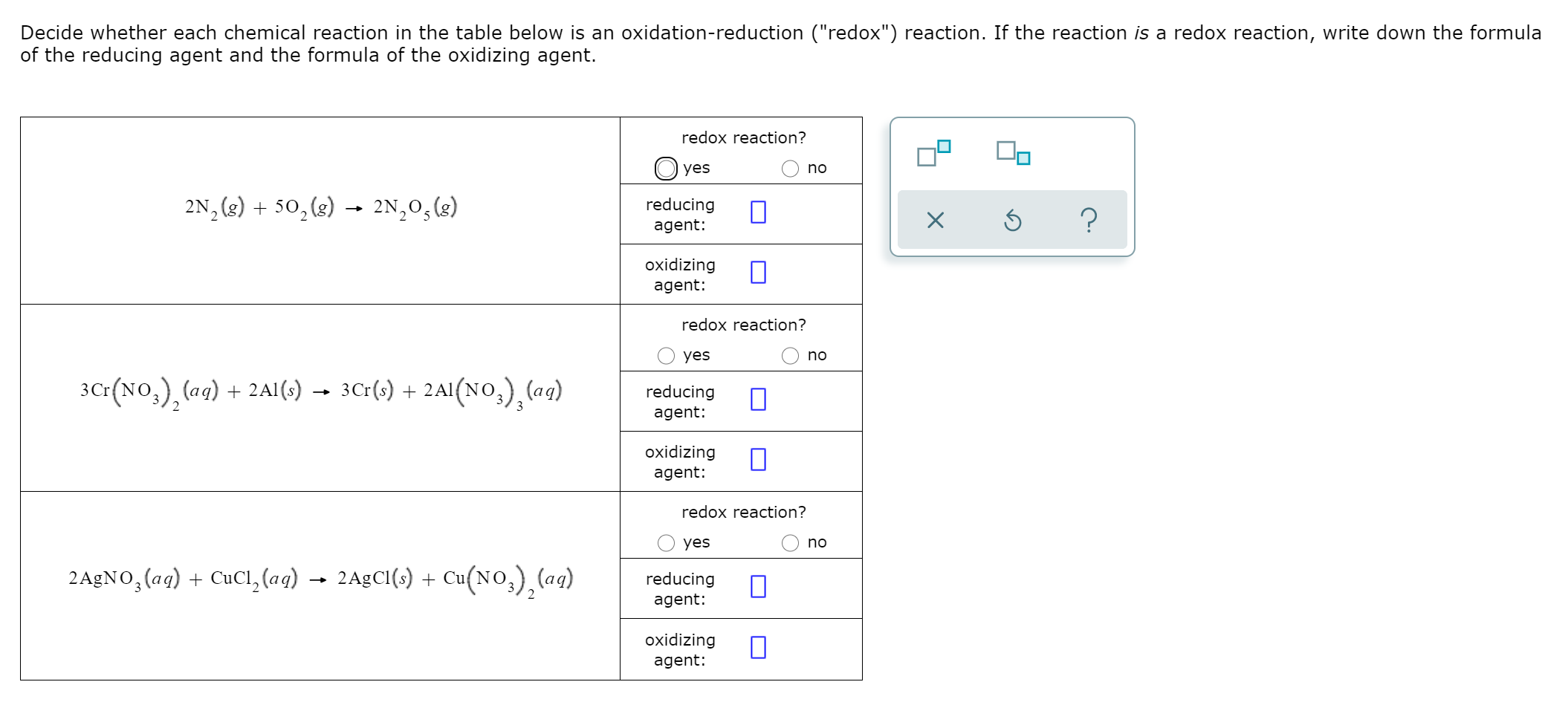Decide whether each chemical reaction in the table below is an oxidation-reduction ("redox") reaction. If the reaction is a redox reaction, write down the formula of the reducing agent and the formula of the oxidizing agent. redox reaction? yes no 2N (8) + 50, (8) + 2N,0, (8) reducing agent: Х 5 ? oxidizing agent: redox reaction? yes no 3Cr(NO3), (aq) + 2A1(s) — 3Cr(s) + 2A1(NO3), (ag) reducing agent: oxidizing agent: redox reaction? yes no 2 AgNO3(aq) + CuCl, (aq) 2 AgCl(s) + Cu(NO3), (aq) reducing agent: oxidizing agent:
Show transcribed image text

This Homework Help Question: "Decide whether each chemical reaction in the table below is an oxidation-reduction ("redox") reaction. If the..." No answers yet.

We need 10 more requests to produce the answer to this homework help question. Share with your friends to get the answer faster!

0 /10 have requested the answer to this homework help question.

Once 10 people have made a request, the answer to this question will be available in 1-2 days.
All students who have requested the answer will be notified once they are available.
##### Add Answer of: Decide whether each chemical reaction in the table below is an oxidation-reduction ("redox") reaction. If the...
Similar Homework Help Questions
• ### Problem Page Decide whether each chemical reaction in the table below is an oxidation-reduction ("redox") reaction....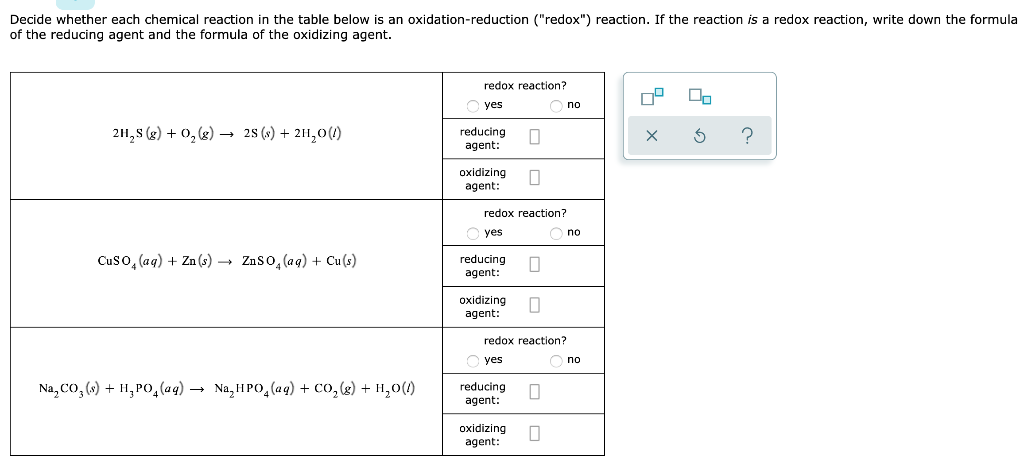Problem Page Decide whether each chemical reaction in the table below is an oxidation-reduction ("redox") reaction. If the reaction is a redox reaction, write down the formula of the reducing agent and the formula of the oxidizing agent. Decide whether each chemical reaction in the table below is an oxidation-reduction ("redox") reaction. If the reaction is a redox reaction, write down the formula of the reducing agent and the formula of the oxidizing agent. redox reaction? yes no 2H, \$...

• ### Decide whether each chemical reaction in the table below is an oxidation-reduction ("redox") reaction. If the...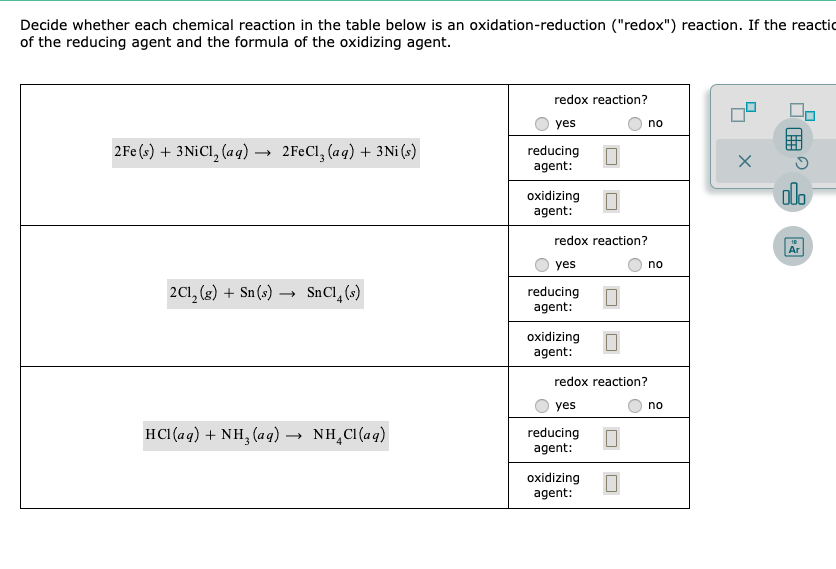Decide whether each chemical reaction in the table below is an oxidation-reduction ("redox") reaction. If the reactic of the reducing agent and the formula of the oxidizing agent. redox reaction? yes no 2 Fe(s) + 3NICI, (aq) — 2FeCl, (aq) + 3Ni (s) reducing agent: oxidizing agent: redox reaction? O yes no 201, (g) + Sn(s) — SnCI, (s) reducing agent: oxidizing agent: 0 redox reaction? yes no HCl(aq) + NH, (aq) → NH,Cl(aq) reducing agent: oxidizing agent:

• ### Decide whether each chemical reaction in the table below is an oxidation-reduction ("redox") reaction. If the...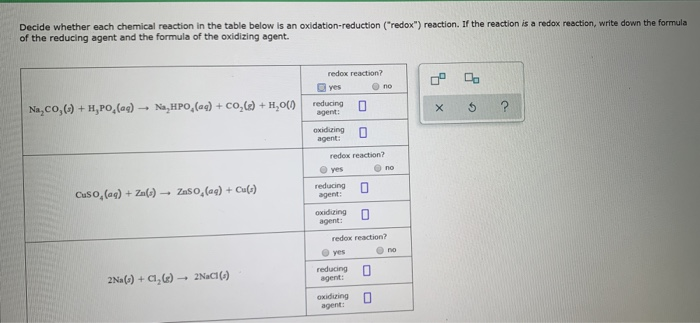Decide whether each chemical reaction in the table below is an oxidation-reduction ("redox") reaction. If the reaction is a redox reaction, write down the formula of the reducing agent and the formula of the oxidizing agent. redox reaction? yes no reducing Na,CO,C.) + H,PO, (ag) - N,HPO,() + COC) + H,01 XS ? egn agent: redox reaction? yes no reducing Cuso, (ag) + Zu() - 250 (0) + () 0 agent U redox reaction? yes no reducing 0 2Na(s) +...

• ### Specify which of the following are oxidation-reduction reactions, and if it is, identify the oxidizing agent,...Specify which of the following are oxidation-reduction reactions, and if it is, identify the oxidizing agent, the reducing agent, the substance being oxidized, and the substance being reduced. If it is not, select No and leave the following boxes blank, Express your answers as a chemical formulas. Omit states-of- matter a. CH (9) + 2O (9) + CO (9) + 2H4O(g) Redox? Oxidizing Agent Reducing Agent Substance Oxidized Substance Reduced Yes b. 2 AgNO3(aq) + Mg(8) + Mg(NO3), (aq) +...

• ### O CAL REACTIONS Identifying addizing and reducing agents Decide whether each chemical reaction in the table...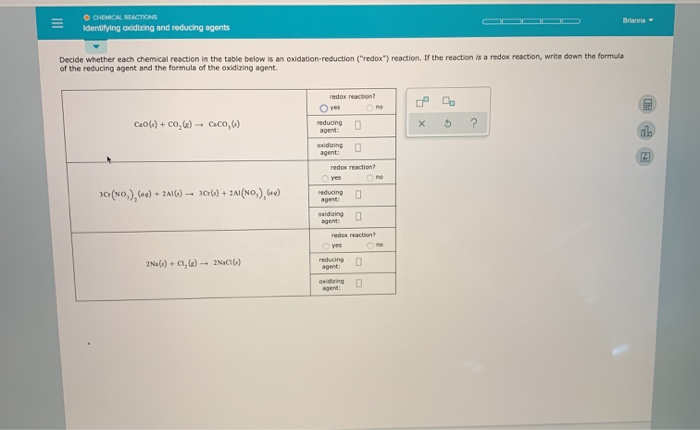O CAL REACTIONS Identifying addizing and reducing agents Decide whether each chemical reaction in the table below is an oxidation-reduction ("redox") reaction. If the reaction is a redox reaction, write down the formula of the reducing agent and the formula of the oxidizing agent. yes no Cao(s) + CO.) → C.CO,6) reducing 0 ? redox reaction? 30(NO), (v) +2010) -- 366) + 2A(NO). ) edung 0 de reaction? 2Na(s) +0,6) - 2006)

• ### O CEMICAL REACTIONS Identifying aiddizing and reducing agents Brianna Decide whether each chemical reaction in the...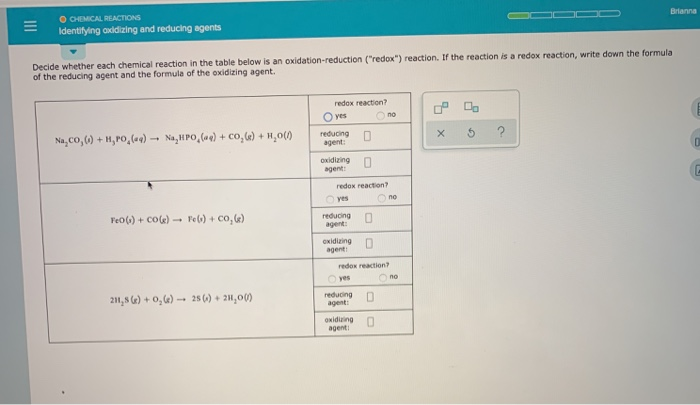O CEMICAL REACTIONS Identifying aiddizing and reducing agents Brianna Decide whether each chemical reaction in the table below is an oxidation-reduction ("redox") reaction. If the reaction is a redox reaction, write down the formula of the reducing agent and the formula of the oxidizing agent. redox reaction? O yes no reducing agent: N.CO,W) + H,PO,(-4) - NHPO(-) + CO, 6) + H2001 x 5 ? oxidang agent: redox reaction yes no FCO (1) + COG) - FW) +0,6) reducing exiding...

• ### oxidation-reduction reactions

Specify which of the following are oxidation-reduction reactions, and identify the oxidizing agent, the reducing agent, the substance being oxidized, and the substancebeing reduced. (Type your answer using the format [Ca]2+ for Ca2+. If the reaction is not an oxidation-reduction reaction, type none in the answer boxes for theoxidizing agent, the reducing agent, the substance being oxidized, and the substance being reduced.)(a) Cu(s) + 2 Ag+(aq) 2 Ag(s) + Cu2+(aq)oxidation-reduction reaction not oxidation-reduction reaction .oxidizing agentreducing agentsubstance being oxidizedsubstance being...

• ### Reference Specify which of the following are oxidation-reduction reactions, and if it is, identify the oxidizing...Reference Specify which of the following are oxidation-reduction reactions, and if it is, identify the oxidizing agent, the reducing a oxidized, and the substance being reduced. If it is not, select No and leave the following boxes blank. Express your ans states-of-matter Cu(s) + 2Ag' (aq) + 2 Ag(s) + Cu?+ (aq) Redox? Oxidizing Agent Reducing Agent Substance Oxidized Substance Reduced b HCl(g) + NH3 (g) - NH4Cl(8) pt Redox? Oxidizing Agent Reducing Agent Substance Oxidized Substance Reduced pl pt...

• ### reduction and Oxidation - half-reactions

The chemical reaction is a spontaneous reaction in which an electrontransfer is taking place and it is called a reduction-oxidation or redox reaction:Cu2+ + Zn (s) ---> Cu (s) + Zn2+The non-spontaneous reaction, i.e., the redox reaction that did not occur, is:Cu (s) + Zn2+ ---> Cu2+ + Zn (s)SO....A. What half-reactions (one a reduction, the other an oxidation) can be written for this redox reaction?B. How many electrons are being transferred from Zn (s) to Cu2+?C. Which is the...

• ### Classify the redox reactions (combination, decomposition, or displacement) and total net ionic...

Classify each of the following redox reactions as a combination, decomposition, or displacement reaction, give a balanced molecular equation for each, as well as totaland net ionic equations for parts (b) and (c), and identify the oxidizing and reducing agents. (Type your answer using the format H2O for H2O and (NH4)2CO3 for(NH4)2CO3. Do not include state symbols in the answers. Use the lowest possible coefficients. Enter 0 if necessary. Do not leave any box blank.)(a) S(s) + O2(g) SO2(g)combination decomposition...

Free Homework App